Next: The third-order central scheme Up: Central schemes in one-space Previous: Central schemes in one-space

In this section we overview the construction of high-resolution central schemes in one-space dimension. We begin with the reconstruction of the second-order, non-oscillatory Nessyahu and Tadmor (NT) scheme, . To approximate solutions of (1.2.1), we introduce a piecewise-linear approximate solution at the discrete time levels,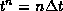, based on linear functions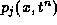which are supported at the cells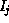(see Figure 1.3.3),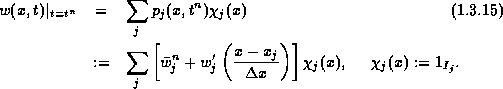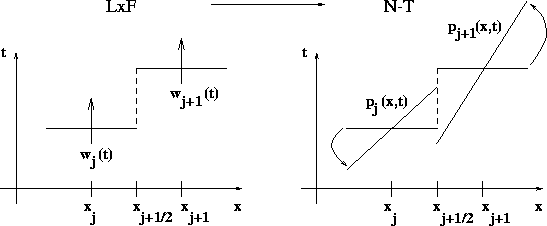Figure 1.3.3: The second-order reconstruction

Second-order of accuracy is guaranteed if the discrete slopes approximate the corresponding derivatives,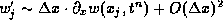. Such a non-oscillatory approximation of the derivatives is possible, e.g., by using built-in non-linear limiters of the form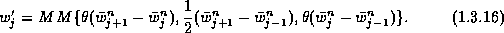Here and below,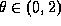is a non-oscillatory limiter and MM denotes the Min-Mod function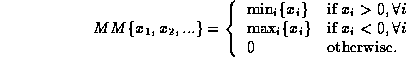An exact evolution of w, based on integration of the conservation law over the staggered cell,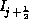, then reads, (1.2.9)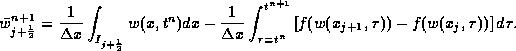The first integral is the staggered cell-average at time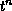,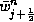, which can be computed directly from the above reconstruction,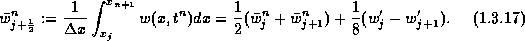The time integrals of the flux are computed by the second-order accurate mid-point quadrature rule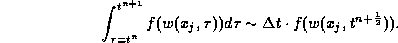Here, the Taylor expansion is being used to predict the required mid-values of w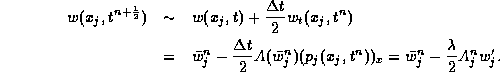In summary, we end up with the central scheme, , which consists of a first-order predictor step,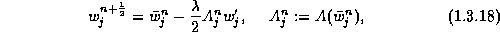followed by the second-order corrector step, (1.2.12),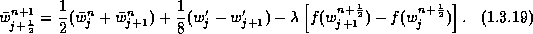The scalar non-oscillatory properties of (1.3.18)-(1.3.19) were proved in , , including the TVD property, cell entropy inequality,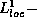error estimates, etc. Moreover, the numerical experiments, reported in , , , , , , , , with one-dimensional systems of conservation laws, show that such second-order central schemes enjoy the same high-resolution as the corresponding second-order upwind schemes do. Thus, the excessive smearing typical to the first-order LxF central scheme is compensated here by the second-order accurate MUSCL reconstruction.

In figure 1.3.4 we compare, side by side, the upwind ULT scheme of Harten, , with our central scheme (1.3.18)-(1.3.19). The comparable high-resolution of this so called Lax's Riemann problem is evident.

At the same time, the central scheme (1.3.18)-(1.3.19) has the advantage over the corresponding upwind schemes, in that no (approximate) Riemann solvers, as in (1.2.7), are required. Hence, these Riemann-free central schemes provide an efficient high-resolution alternative in the one-dimensional case, and a particularly advantageous framework for multidimensional computations, e.g., , , . This advantage in the multidimensional case will be explored in the next section. Also, staggered central differencing, along the lines of the Riemann-free Nessyahu-Tadmor scheme (1.3.18)-(1.3.19), admits simple efficient extensions in the presence of general source terms, , and in particular, stiff source terms, . Indeed, it is a key ingredient behind the relaxation schemes studied in .

It should be noted, however, that the component-wise version of these central schemes might result in deterioration of resolution at the computed extrema. The second-order computation presented in figure 1.3.2 below demonstrates this point. (this will be corrected by higher order central methods). Of course, this - so called extrema clipping, is typical to high-resolution upwind schemes as well; but it is more pronounced with our central schemes due to the built-in extrema-switching to the dissipative LxF scheme. Indeed, once an extrema cell,, is detected (by the limiter), it sets a zero slope,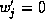, in which case the second-order scheme (1.3.18)-(1.3.19) is reduced back to the first-order LxF, (1.2.14).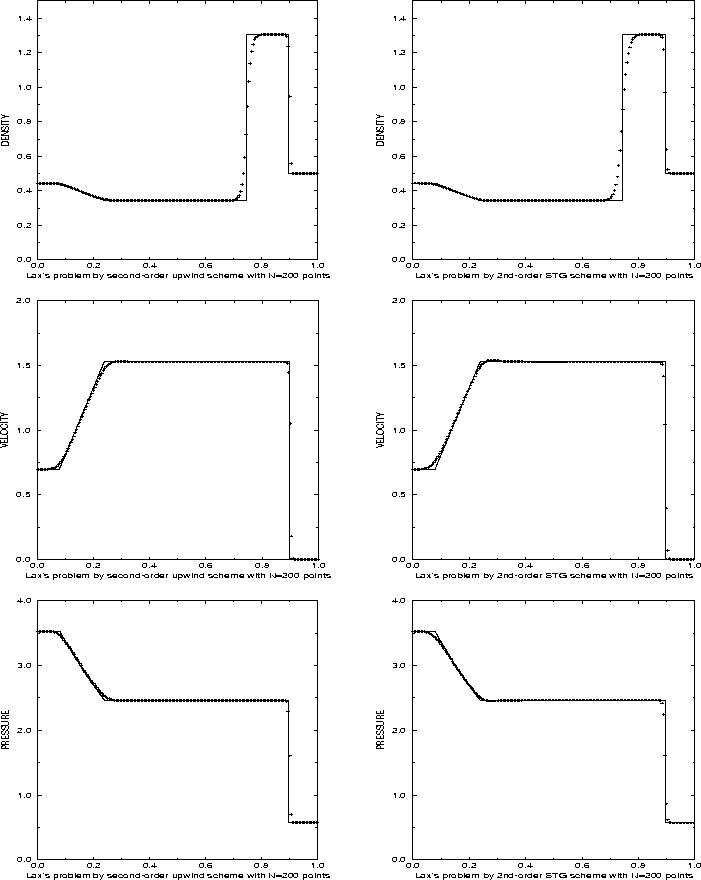Figure 1.3.4: 2nd order: central (STG) vs. upwind (ULT) -- Lax's Riemann problemNext: The third-order central scheme Up: Central schemes in one-space Previous: Central schemes in one-space